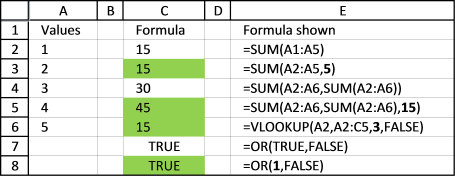Author: Oscar Cronquist Article last updated on April 23, 2017

Sometimes you need to find formulas containing literals (hard coded values) in a workbook.

I found this excellent UDF in David Hager´s Excel Experts E-letter (EEE) on J Walkenbach’s website.

```Function CellUsesLiteralValue(Cell As Range) As Boolean
If Not Cell.HasFormula Then
CellUsesLiteralValue = False
Else
CellUsesLiteralValue = Cell.Formula Like "*[=^/*+-/()><, ]#*"
End If
End Function
```

---How can I locate cells containing formulas with literal values?---

Use the UDF as your conditional formatting formula.
It accepts a single cell as an argument. It returns True if the cell's formula contains an operator followed by a numerical digit. In other words, it identifies cells that have a formula which contains a literal numeric value. You can test each cell in the range, and highlight it if the function returns True.
by John Walkenbach and John Green

### It is a small efficient UDFThe UDF with conditional formatting highlights cells containing formulas with literals, column C. You can download an excel file at the end of this post, if you are interested in this technique.
I have bolded all literal values in the formulas, column E. It seems to work with nested formulas also. Boolean values can be expressed as TRUE/FALSE but also 1/0 in excel. Remember, some formulas are built to have hard coded arguments, like col_index_num in VLOOKUP function.

### I have a large workbook and I am in a hurry!

The authors want you to use it as conditional formatting formula to spot cells containing formulas with literal values, that can be tedious work with a large workbook. I would like to show you how to use the udf to build a list of all cells containing literal values, from an entire workbook.

This picture shows you what my macro below returns, first a new sheet is inserted. It is then populated with links to all cells containing formulas with literals and their corresponding formulas.I have reused some parts of Rick Rothstein´s macro.

```Sub FindLiteralsInWorkbook()
Dim C As Range, A As Range, Addresses As String, i As Single
Dim cell As Range
Set x = ActiveSheet
x.Range("B1") = "Formula"
i = 1
For Each sh In ActiveWorkbook.Worksheets
On Error Resume Next
Set C = sh.Cells.SpecialCells(xlConstants)
If C Is Nothing Then
Set C = sh.Cells.SpecialCells(xlFormulas)
Else
Set C = Union(C, sh.Cells.SpecialCells(xlFormulas))
End If
For Each A In C.Areas
For Each cell In A
If CellUsesLiteralValue(cell) = True Then
i = i + 1
Address:=ActiveWorkbook.Path & "\" & ActiveWorkbook.Name, _
x.Range("B" & i) = "'" & cell.Formula
End If
Next cell
Next A
On Error GoTo 0
Next sh
x.Columns("A:E").AutoFit
End Sub
```

### How to use this macro

1. Copy UDF CellUsesLiteralValue and macro FindLiteralsInWorkbook
2. Go to VB Editor (Alt + F11)
3. Go to the menu and click Insert
4. Click Module
5. Paste UDF and macro to your workbook´s code module